# NCERT Solution Exercise 7.2 Class 10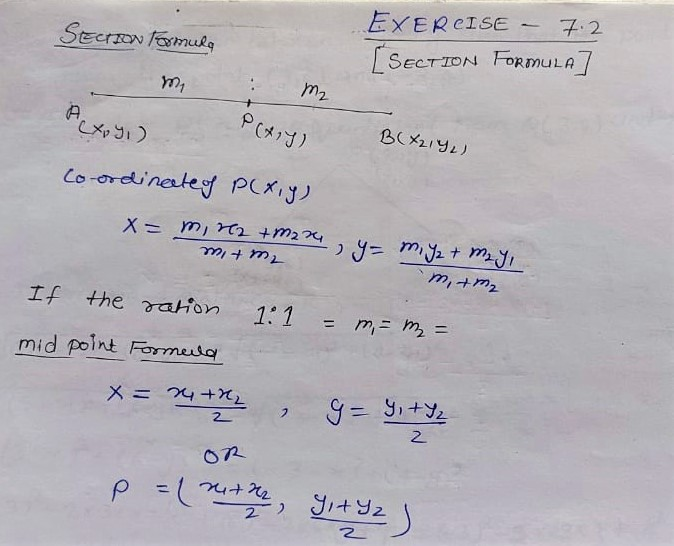Q.1 Find the coordinates of the point which divides the join of (-1,7) and (4,-3) in the ratio 2:3.

Solution: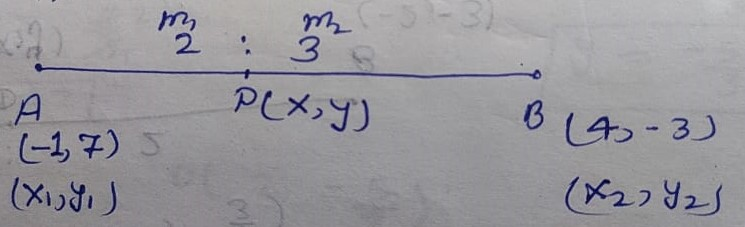Let A(-1,7) and B(4, -3) and Ratio m1 : m2 = 2 : 3

fig

By Section Formula

Coordinate of P(x, y)

x = (m1x2 + m2x1)/m1+m2 ; y = (m1y2 + m2y1)/m1+m2

⇒ x = (2×4 + 3×-1)/ 2+3 ; y = (2×-3 + 3×7)/2+3

⇒ x = (8 – 3)/5 ; y = (-6 + 21)/5

⇒ x = (5)/5 ; y = (15)/5

⇒ x = 1 ; y = 3

Hence co-ordinate of division point P = (1,3)

Q.2 Find the coordinates of the points of trisection of the line segment joining (4, -1) and (-2,-3).

Solution: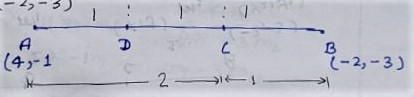By Section formula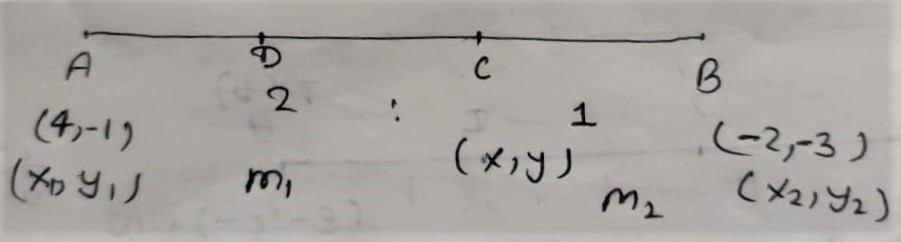Coordinate of C(x, y)

x = (m1x2 + m2x1)/m1+m2; y = (m1y2 + m2y1)/m1+m2

⇒ x = (2×-2+1×4)/(2+1) ; y = (2×-3 + 1×-1)/(2+1)

⇒ x = (-4 + 4)/3 ; (-6 -1)/3

⇒ x = (0)/3 ; (-7)/3

⇒ x = 0 ; -7/3

C (0, -7/3)

Coordinate of D (x, y)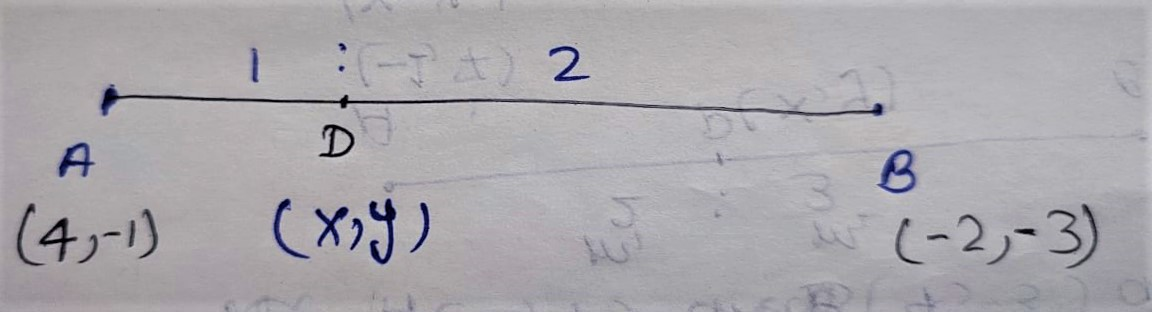x = (m1x2 + m2x1)/m1+m2;  y = (m1y2 + m2y1)/m1+m2

x = (1×-2 + 2×4)/1+2 ; y = (1×-3 + 2×-1)/1+2

⇒ x = (-2 +8)/3 ; y = (-3 -2)/3

⇒ x = 6/3 ; y = (-5)/3

⇒ x = 2 ;

D =(2, -5/3)

Hence co-ordinate of trisection point C (0,-7/3), D (2,-5/3)

Q.4 Find the ratio in which the line segment joining the points (-3,10) and (6, -8) is divided by (-1,6).

Solution: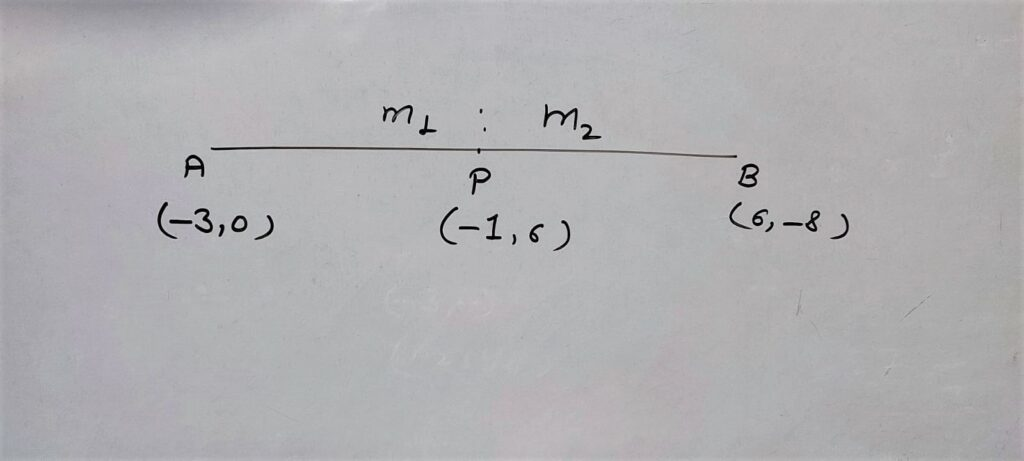Let Point A(-3,10), and B(6,-8) is divided by P(-1,6) in ration m1: m

By division/section Formula

x = (m1x2 + m2x1)/m1+m2

⇒ -1 = (m16 + m-3)/m1+m2

⇒ -1(m1+m2) = (6m1 -3 m2)

⇒ -m1-m2 = 6m1 -3 m2

⇒ -m-6m= -3 m2 -m2

⇒ -7m1 = – 4m2

⇒ m1/m2 = -4/-7

⇒ m1/m2 = 4/7

⇒ m1:m2 = 4:7

Hence the ratio = 2 : 7

Note: You can also find the ratio by y coordinate .

Q.5 Find the ration in which the line segment joining A(1, -5) and B(-4, 5) is divided by the x- axis. Also find the coordinates of the point of division.

Solution:

Let the point on x-axis be P(x, 0)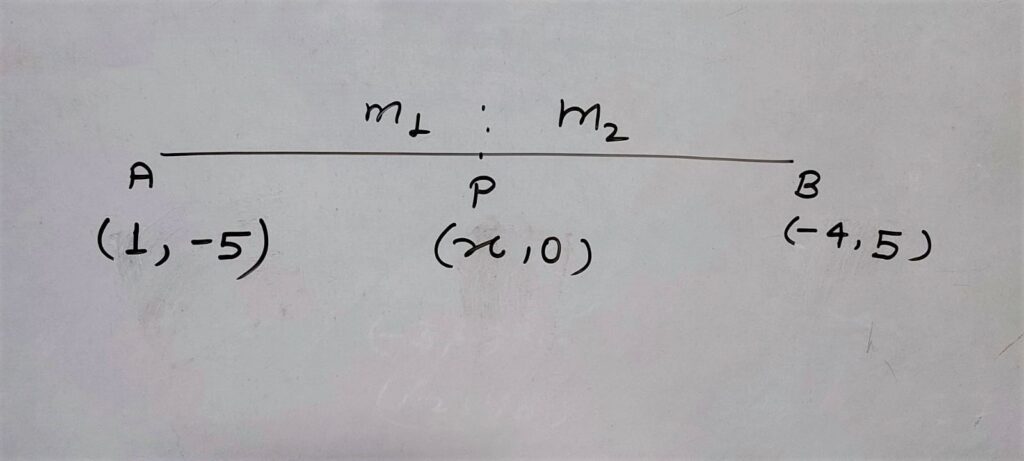By section formula

y = (m1y2 + m2y1)/m1+m2

⇒ 0 =  (m1y2 + m2y1)/m1+m2

⇒ 0 = (m1×5 + m2×-5)/m1+m2

⇒ 0 = (5m1 – 5 m2)/ (m1+m2)

⇒ 0(m1+m2) = 5m1 – 5 m2

⇒ 0 = 5m1 – 5 m2

⇒ 5 m2  = 5m1

⇒ 5/5 = m1/ m2

⇒ 1 : 1 = m1 : m2

∴ division point p is mid point

x = (m1x2 + m2x1)/m1+m2

⇒ x = (1×-4 + 1×1)/1+1

⇒ x = (-4 + 1)/2

⇒ x = -3/2

Hence co-ordinate on x-axis = (-3/2,0)

Q.6 If (1,2), (4, y), (x, 6) and (3, 5) are the vertices of a parallelogram taken in order, find x and y.

Solution:

In parallelogram ABCD Diagonal AC and BD are bisect each other at point O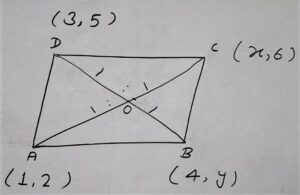Therefore coordinate of O by A And B = C-ordinate of O by B and D are same.

Now

Coordinate of O by A (1,2) and C (x, 6)

By Mid -point formula

O = (x1+x2/2 , y1+y2/2)

O =(1+x/2 , 2+6/2)

O = (1+x/2 , 8/2)

O =  (1+x/2, 4)

Similarly co-ordinate of ) by Diagonal B(4,y) and D (3,5)

O = (x1+x2/2 , y1+y2/2)

O = (4+3/2 , y+5/2 )

O = (7/2 , y+5/2)

By comparing

1+x / 2 = 7/2    ;  4 = y+5/2

⇒ 1+ x = 7       ; 4×2 = y+5

⇒ x = 7-1         ; 8 = y+5

⇒ x = 6             ; 8-5 = y

⇒ x = 6             ; 3 = y

Hence x = 6 and y = 3

Q.7 Find the coordinates of a point A, where AB is the diameter of a circle whose Centre is (2, -3) and B is (1, 4).

Solution: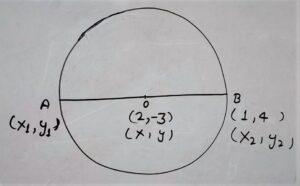Center O divides Diameter AB in equal Ratio

∴ By mid – point formula

x =  (x1+x2)/2  ;

⇒ 2 = (x1+1)/2 ;

⇒ 2×2 = x1+1

⇒ 4 =  x1+1

⇒ 4 – 1 = x1

⇒ 3 = x1

y = ( y1+y2)/2

⇒ -3  =  (y1+4)/2

⇒ -3×2  =  y1+4

⇒ -6 – 4  =  y1

⇒ -10 = y1

Hence Co-ordinate of A (3, -5)

Q8. If  A and B are (-2, -2) and (2, -4), respectively, find the coordinates of P such that AP = 3/7 AB and P lies on the line segment AB.

Solution: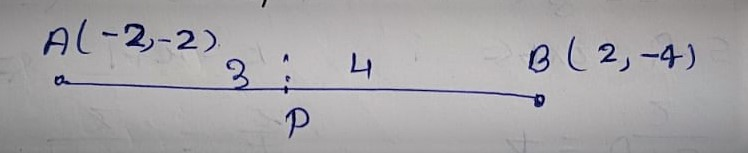AP = 3/7 AB

⇒ AP/AB = 3/7

PB = AB – AP

PB = 7 – 3 = 4

Let the co-ordinate of P(x, y)

By Section Formula

x = (m1x2 + m2x1)/(m1+m2)               ;  y = (m1y2 + m2y1)/(m1+m2)

x =  (3×2 + 4×-2)/(3+4)               ; y = (3×-4 + 4×-2)/(3+4)

x = (6 – 8)/7                                  ; y = (-12-8)/7

x = -2/7                                       ; y =-20/7

Hence Co-ordinate of (-2/7 , -20/7)

Q.9 Find the coordinates of the points which divide the line segment joining A(-2, 2) and B(2, 8) into four equal parts.

Solution: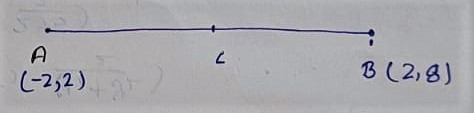Let mid-point of A(-2, 2) and B(2, 8) is C

C = ( x1+x2 /2  , y1+y2 /2 )

C = ( -2+ 2 /2  , 2+8 /2 )

C = ( 0 /2  , 10 /2 )

C = (0, 5)

Let Mid-point of A(-2,2) and C (0,5) be DD = ( x1+x2 /2  , y1+y2 /2 )

D = ( -2+0/2 , 2+5/2 )

D = (-2/2 , 7/2)

D = (-1 , 7/2)

Let mid-point of C(0, 5) and B (2, 8) be  E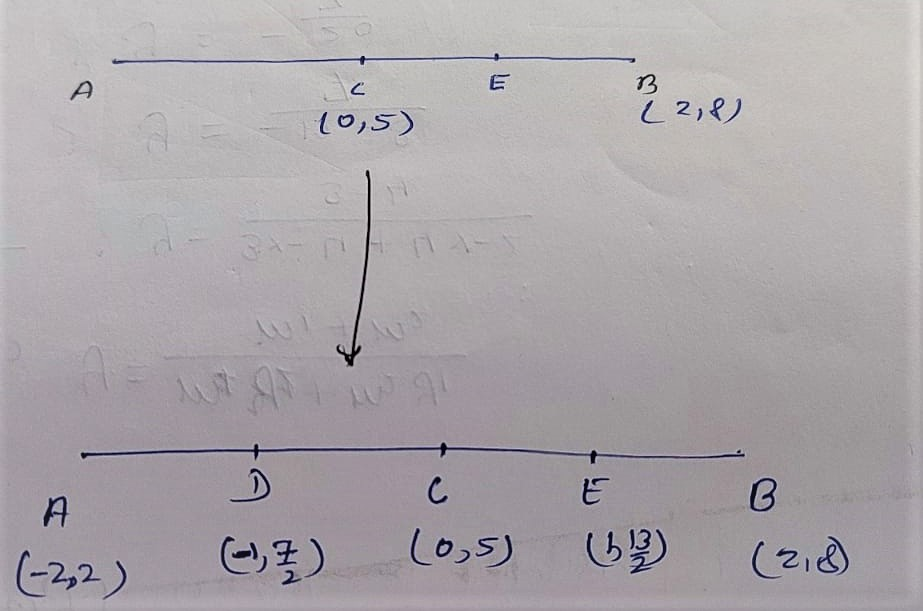E = ( x1+x2 /2  , y1+y2 /2 )

E = ( 0+2/2 , 5+8/2 )

E = ( 2/2 , 13/2 )

E = (1, 13/2)

Q.10 Find the area of a Rhombus if its vertices are (3, 0),(4, 5),(-1,4) and (-2,-1) taken in order.

Solution: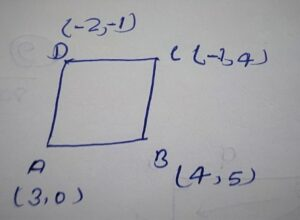Area of Rhombus = 1/2 (product of diagonals)

⇒ Area of Rhombus = 1/2 ×AC ×BD

By Distance formula

AC = √(x2-x1)² + (y2-y1)²

⇒ A C = √(x2 – x1)² + (y2 – y1

⇒ A C = √(-1 – 3)² + (4 – 0)²

⇒ A C = √(-4)² + (4)²

⇒ A C = √16+16

⇒ A C = √32

⇒ A C = 16√2

B D = √(x2-x1)² + (y2-y1)²

⇒  B D = = √(-2 – 4)² + (-1-5)²

⇒  B D = = √(-6)² + (-6)²

⇒  B D = = √36 + 36

⇒  B D = √72

⇒  B D = 6√2

Area of Rhombus = 1/2 ×4√2×6√2

⇒ Area of Rhombus = 24×2/2

⇒ Area of Rhombus = 24 square units.